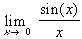#Interactive Real Analysis

Next | Previous | Glossary | Map

## 6.5. Differentiable Functions

### Examples 6.5.16(a):

FindYour browser can not handle Java applets L'Hospital's Rule applies directly in this case. If g(x) = sin(x) and f(x) = x, then g'(x) = cos(x) and f'(x) = 1. Hence, by l'Hospital's rule, we have:= cos(0) / 1 = 1
Next | Previous | Glossary | Map